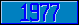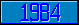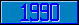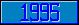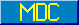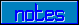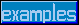## \$%TAN

### M[UMPS] by Example

```
2-Jul–96, 6:54:35

Routine Save for Trigonometry Library function \$%TAN^MATH

;

; Unless otherwise noted, the code below

; was approved in document X11/95–12

;

; If corrections have been applied,

; first the original line appears,

; with three semicolons at the beginning of the line.

;

; Then the source of the correction is acknowledged,

; then the corrected line appears, followed by a

; line containing three semicolons.

;

TAN(X,PREC) ;

New L,LIM,K,S,SIGN,VALUE

;

;;; Set:X[":" X=\$%DMSDEC^MATH(X,12)

; Winfried Gerum (8 June 1995)

;    Comment: The official description does not mention than

;             the function may also be called with the first

;             parameter in degrees, minutes and seconds.

Set:X[":" X=\$%DMSDEC^MATH(X)

;;;

;

Set PREC=\$Get(PREC,11)

Set X=X#(2*\$%PI^MATH())

Set (VALUE,L)=X,SIGN=–1

Set LIM=\$Select((PREC+3)'>11:PREC+3,1:11),@("LIM=1E-"_LIM)

For K=3:2 Do  Quit:(\$Translate(L,"-")<LIM)  Set SIGN=SIGN*–1

. Set L=L/(K–1)*X/K*X,VALUE=VALUE+(SIGN*L)

. Quit

Set S=VALUE

Set X=X#(2*\$%PI^MATH())

Set (VALUE,L)=1,SIGN=–1

Set LIM=\$Select((PREC+3)'>11:PREC+3,1:11),@("LIM=1E-"_LIM)

For K=2:2 Do  Quit:(\$Translate(L,"-")<LIM)  Set SIGN=SIGN*–1

. Set L=L*X*X/(K–1*K),VALUE=VALUE+(SIGN*L)

. Quit

If 'VALUE Quit "INFINITE"

Quit S/VALUE

;===

;

;

;

```